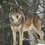# Euler's identity

Euler's identity: $e^{i\pi} =-1$

A different approach to derive this equation without using series

$\displaystyle \int_{0} ^{x} \frac{1}{1+x^{2}} dx =\arctan x$

$\displaystyle \frac{1}{1+x^{2}} =\frac{1}{2} \left(\frac{1}{1+xi} +\frac{1}{1-xi}\right)$

$\displaystyle \int_{0}^{x} \frac{1}{2} \left(\frac{1}{1+xi} +\frac{1}{1-xi}\right) dx = \frac{1}{2i} \left(\ln\left(1+xi\right)-\ln\left(1-xi\right)\right)$

$\frac{1}{2i} \left(\ln\left(1+xi\right)-\ln\left(1-xi\right)\right) =\arctan x$

We know $\arctan 1 =\frac{\pi}{4}$

$\frac{1}{2i} \left(\ln\left(1+i\right)-\ln\left(1-i\right)\right) =\frac{\pi}{4}$

$\left(\ln\left(1+i\right)-\ln\left(1-i\right)\right) =\frac{\pi\times i}{2}$

$\displaystyle \ln\left(\frac{1+i}{1-i}\right) =\frac{\pi\times i}{2}$

$\displaystyle\ln\left(\frac{\left(1+i\right)^{2}}{2}\right) =\frac{\pi\times i}{2}$

$\displaystyle\ln\left(\frac{1+2i -1}{2}\right) =\frac{\pi\times i}{2}$

$\displaystyle\ln\left(i\right) =\frac{\pi\times i}{2}$

$\displaystyle \exp^{\ln\left( i \right)} =\exp^{\frac{i \pi}{2}}$

$\displaystyle i=e^{\frac{i \pi}{2}}$

square both sides to obtain $-1 =e^{i\pi}$Note by Amal Hari
1 year, 6 months ago

This discussion board is a place to discuss our Daily Challenges and the math and science related to those challenges. Explanations are more than just a solution — they should explain the steps and thinking strategies that you used to obtain the solution. Comments should further the discussion of math and science.

When posting on Brilliant:

• Use the emojis to react to an explanation, whether you're congratulating a job well done , or just really confused .
• Ask specific questions about the challenge or the steps in somebody's explanation. Well-posed questions can add a lot to the discussion, but posting "I don't understand!" doesn't help anyone.
• Try to contribute something new to the discussion, whether it is an extension, generalization or other idea related to the challenge.
• Stay on topic — we're all here to learn more about math and science, not to hear about your favorite get-rich-quick scheme or current world events.

MarkdownAppears as
*italics* or _italics_ italics
**bold** or __bold__ bold
- bulleted- list
• bulleted
• list
1. numbered2. list
1. numbered
2. list
Note: you must add a full line of space before and after lists for them to show up correctly
paragraph 1paragraph 2

paragraph 1

paragraph 2

[example link](https://brilliant.org)example link
> This is a quote
This is a quote
    # I indented these lines
# 4 spaces, and now they show
# up as a code block.

print "hello world"
# I indented these lines
# 4 spaces, and now they show
# up as a code block.

print "hello world"
MathAppears as
Remember to wrap math in $$ ... $$ or $ ... $ to ensure proper formatting.
2 \times 3 $2 \times 3$
2^{34} $2^{34}$
a_{i-1} $a_{i-1}$
\frac{2}{3} $\frac{2}{3}$
\sqrt{2} $\sqrt{2}$
\sum_{i=1}^3 $\sum_{i=1}^3$
\sin \theta $\sin \theta$
\boxed{123} $\boxed{123}$

## Comments

There are no comments in this discussion.

×

Problem Loading...

Note Loading...

Set Loading...